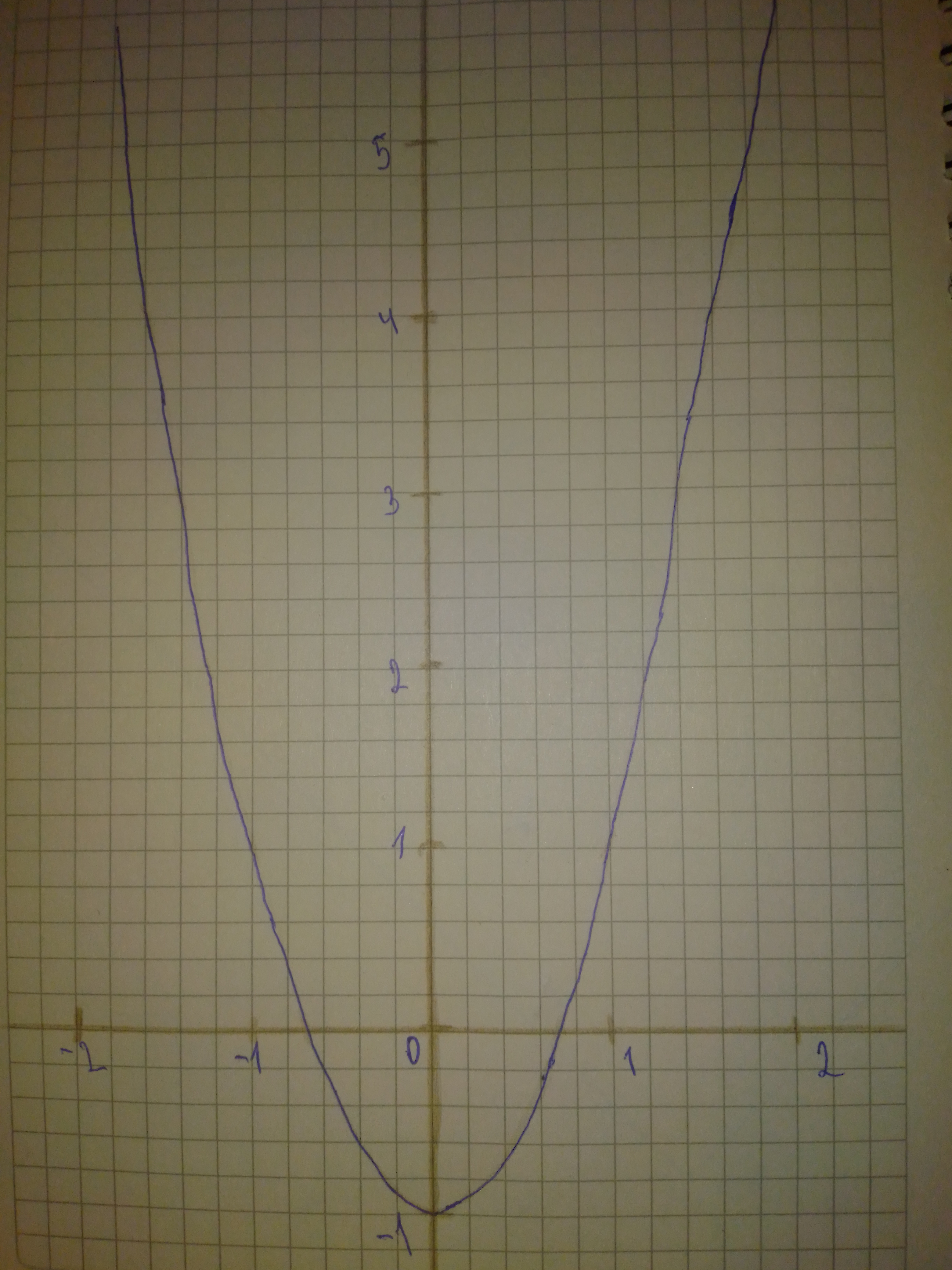Sketch a graph of the function. Use transformations of functions when ever possible. f(x)=2x^{2}-1FobelloE 2020-12-01 Answered
Sketch a graph of the function. Use transformations of functions when ever possible.
$$\displaystyle{f{{\left({x}\right)}}}={2}{x}^{{{2}}}-{1}$$

• Questions are typically answered in as fast as 30 minutes

Solve your problem for the price of one coffee

• Math expert for every subject
• Pay only if we can solve itjoshyoung05M

Step 1
Shown is the graph of $$\displaystyle{f{{\left({x}\right)}}}={2}{x}^{{{2}}}-{1}$$
x is on the horizontal axis and y is on the vertical axis Step 2